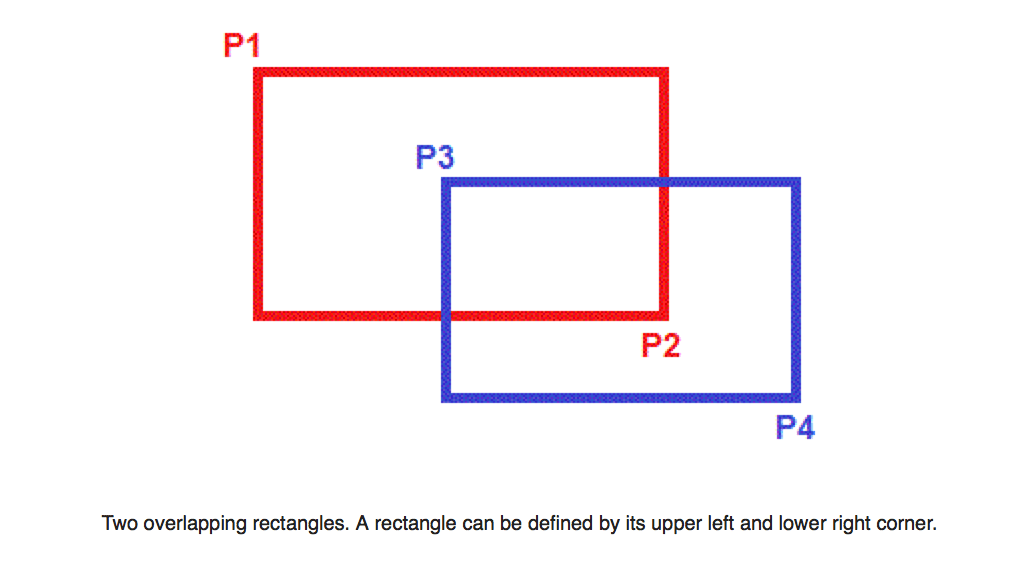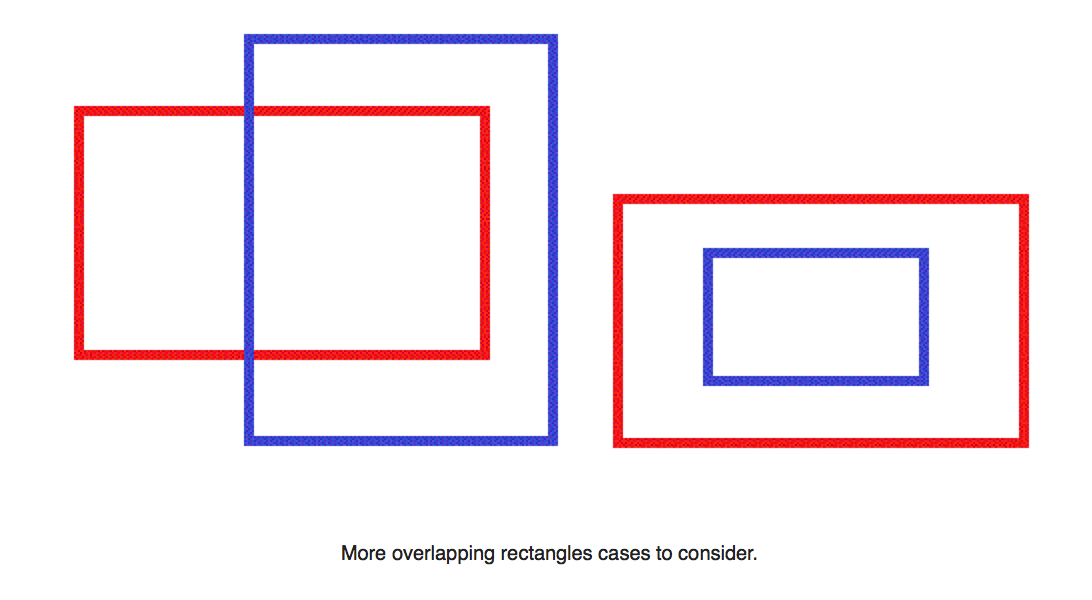# Determine if 2 rectangles overlap

By | July 17, 2015

Question: You are given two axis-aligned rectangles. You have to determine if these rectangles overlap each other or not.
Rectangle 1 : P1 (x, y), P2 (x,y)
Rectangle 2 : P3 (x,y), P4 (x,y)

In this problem statement, we are given co-ordinates for 2 rectangles and we have to determine if they overlap or not. In a way we can say that our use case looks like this.At the first glance, the problem seems to look very complicated. If you are coming up with a complicated set of conditionals, you might think too hard. There is an easier way. Try to think in the opposite direction.

Assume that the two rectangles are given as point (P1, P2) and (P3, P4) respectively. One direct way to attempt this problem is when two rectangles overlap, one rectangle’s corner point(s) must contain in the other rectangle. Do keep in mind of the following cases:When we look at the problem now, it seems to get more complicated because of the 8 conditional statements which seem to be necessary immediately. Can we simplify it further?

YES, by using the negation method.

Do you remember the probability questions where you have to determine the chance such that no black ball comes out of a bag having 7 different color balls? We find the probability of black ball and then subtract it from 1 to get the result.

Similarly, A much easier and better approach would be to think from the opposite. How about asking yourself how the two rectangles cannot overlap each other? Two rectangles do not overlap when one is above/below, or to the left/right of the other rectangle.
So, when we try to workout the expression we get:

```! ( P2.y < P3.y || P1.y > P4.y || P2.x < P3.x || P1.x > P4.x )
```

This can be simplified further using De Morgan’s law:-

```( P2.y <= P3.y && P1.y >= P4.y && P2.x >= P3.x && P1.x <= P4.x )
```

Pretty neat!!!Author: nikoo28

a tech-savvy guy and a design buff...

## 8 thoughts on “Determine if 2 rectangles overlap”

1.Tristan Rentz

This is still fundamentally incorrect! Check your logic. I repeat to you: the negation of ‘less than’ is NOT ‘equal to’, it is ‘greater than or equal to’, so your simplification is wrong. Also think of the implications of your result, ie apply it to your rectangles.. Unless one of the rectangles is flipped on both axes, you will by definition never get a ‘true’. Even when you do, you will only get ‘true’ if the rectangles are exactly the same size and shape, and occupying exactly the same space.
Dude! Look closely. You need to CHECK YOUR WORK and FIX THIS POST!

1.nikoo28 Post author

Hi Tristan,
I apologize for my mistake. I looked into your solution and realized it was the correct way as per some test cases.
The post has now been corrected.

Thanks.

2.Tristan Rentz

Are you sure about that?? From what I can see, the implications of yr result are that the negation of ‘less than’ is ‘equal to’, as opposed to ‘greater than or equal to’. More practically speaking, yr result appears to find rectangles that touch, but do not overlap…

1.nikoo28 Post author

There was a little typo in the post and I have corrected it. If you can list out a test case that fails, I will look into the method.
Thanks

1.Tristan Rentz

Hi Nikoo
Simply put:
! ( P2.y P4.y || P2.x P4.x )
does not simplify to
( P2.y = P3.y && P1.y = P4.y && P2.x = P3.x && P1.x = P4.x )
but does simplify to
( P2.y >= P3.y && P1.y = P3.x && P1.x >= P4.x )
That is to say:
! ( P2.y = P3.y
NOT to:
P2.y = P3.y
Then expand the rest accordingly. If I’m wrong, please explain how.

2.Tristan Rentz

Hey Nikoo

A real issue is: this site modifies one’s text before publishing, and now my latest reply is full of typos, including typos imposed on text that has been copied and pasted.. :(

3.Rafael R. S. Robles

Nice solution, I was thinking the hard way

1.nikoo28 Post author

You are welcome to publish your way of solving the problem.

This site uses Akismet to reduce spam. Learn how your comment data is processed.